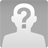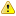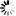#Exploring Fantom DSLsYou are viewing an old version of this page. View the current version.

### Brief intro to DSLs

Fandoc says:

DSLs

DSLs or Domain Specific Languages allow you to embed other languages into your Fantom source code. The syntax for a DSL is:

AnchorType <|...|>

Everything between the <| and |> tokens is considered source code of the DSL itself. The anchor type defines how to the compile the DSL. DslPlugins are registered on the anchor type, and called by the Fantom compiler to translate them into a Fantom expression.

Technically speaking, DSLs are presented as DSL expressions, and it's result type is defined by the anchor type, so that

```  str := Str<|hello, world!|>  // str has type Str
foo := Foo<|bar|> // foo has type Foo
```

Therefore, the most obvious way to use DSL expressions is instantiation of some complex objects. For example, imagine we are writing a library for graph manipulation, so we define classes like Graph, Node, and Edge. See Graphs.fan
and we need to instantiate some graphs for our tests, so we write the code like this:

``` a := Node("a")
b := Node("b")
c := Node("c")
d := Node("d")
ab := Edge(a, b)
bc := Edge(b, c)
bd := Edge(b, d)
graph := Graph([a,b,c,d], [ab, bc, bd])
```

What a lot of code! Let's use some DSL magic:

```  graph := Graph<|a -> b
b -> c
b -> d|>

```

The DSL plugin for Graph just parses the code between <| and |> (which is accessed via compiler::DslExpr.src) and generates appropriate object creation.

However even such a simple example is quite tricky - to allow our DSL to be used as field initializer, or default parameter value, we need to convert our DSL code to a single expression.

So, before implementing the DSL plugin itself, we need to understand how we can replace our code with a single expression. In this particular example, assuming that Node and Edge classes override equals and hash correctly, it can be done like this:

```Graph(
["a","b","c","d"].map {
Node(it)
},
[
["a","b"],
["b", "c"],
["b", "d"]].map {
Edge(
Node(it.first),
Node(it.last)
)
}
)
```

The complete source code for example above can be found here.
So I thought - nice feature, but rarely needed, also the compiler API operates on a quite low-level, so that even simple constructor invocation requires too many code.

### Beyond the DSL src

Labels:
None